# SAT II Math II : Finding Sides

## Example Questions

2 Next →

### Example Question #51 : Geometry

Given a cube, if the volume is 100 feet cubed, what must be the side?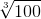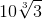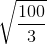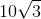Explanation:

Write the formula for the volume of the cube.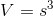To solve for, cube root both sides.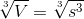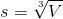Substitute the volume.

The answer is:2 Next →